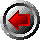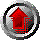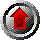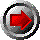PreviousHomeContentsNext

# Chapter 9: Generators

Consider the problem of traversing a list to operate on each of its members. One might write code such as:

```-- Approach 1: tailing
t := L;
while not empty? t repeat {
a := first t;
t := rest t;
f a
}
```

This approach makes good sense for linked lists, but is too expensive for lists represented as arrays. Each iteration would have to allocate a new array in the call to `rest`, leading to O(#L2) storage use where none is really necessary.

```-- Approach 2: indexing
for i in 1..#L repeat {
a := L.i;
f a;
}
```

This approach makes good sense for lists represented as arrays, but is inappropriate for a linked list representation. Each iteration would need to traverse the list from the begining to find the desired element, leading to O(#L2) time where O(#L) should suffice.

Having seen this, consider the problem of writing a generic program to operate on some `FiniteLinearAggregate` structure which might be represented as an array, as a linked list, or in some other way.

How should loops be written to minimize cost in a generic program?

A related problem arises with more sophisticated data structures. Here, the steps to traverse an object can be rather intricate. The code for a loop which traverses two objects in parallel can be extremely difficult and error-prone.

How can loops be written which hide the complexity of data representation?

The answer to both these questions is the same in Aldor, and that is to use generators.

### 9.1 : Using generators in loops

Generators may be used in Aldor to obtain values serially, wherever required. For example: to compute numbers in a sequence; to access the components of a composite structure, such as a list, array or hash table; or to read data from a file.

The simplest way to use a generator in Aldor is with a for iterator on a repeat loop or a collect form:

```#include "aldor"

-- Generators in a for-repeat loop.
import from Generator SingleInteger;
g := generator(1..10);

for i in g repeat { print << i << newline }

-- Generators in a for-collect loop.
import from List SingleInteger;
h := generator(1..10);

l := [ i*i for i in h ];

print << l << newline
```

In fact, this form of using generators is so common, that if the expression E in ``for v in E.'' does not belong to a generator type, then an implicit call is made to an appropriate generator function. This is equivalent to writing ``for v in generator E.''

With this, our example may be written as:

```#include "aldor"

-- Implicit generators in a  for-repeat loop.
import from SingleInteger;

for i in 1..10 repeat { print << i << newline }

-- Implicit generators in a  for-collect loop.
import from List SingleInteger;

l := [ i*i for i in 1..10 ];

print << l << newline
```

In Aldor all for-repeat and for-collect loops are based on generators. There is no built in method to traverse integer ranges, lists or other structures. It is the compiler's responsibility to make the use of generators reasonably efficient.

Generators are regular values in Aldor and, once created, may be passed as arguments to functions which consume them gradually, according to a cooperative scheme.

### 9.2 : Using generators via functions

Sometimes it is desired to use the values in a generator gradually, with some logic that is not naturally expressed as a `for` loop.

One might imagine writing a function, such as the following, to extract elements from a generator one at a time.

```first(g: Generator S): Union(value: S, nil: Pointer) == {
for s in g repeat return s;
nil
}
```

Here the loop body would be evaluated zero or one times, and the function would return `nil` or the first value in the generator. Subsequent calls would extract the remaining values.

The base Aldor library provides a set of functions which can be used in this way, but are somewhat more flexible. To use these functions one must have an include command for ``aldor'' and import from the appropriate generator type, e.g.:

```#include "aldor"
import from Generator T;
...
```

With this, the following functions become available:

• step!: Generator T -> Generator T
• empty?: Generator T -> Boolean
• value: Generator T -> T
• bound: Generator T -> SingleInteger

The function "`step!`" runs the generator code until the next value, or the end of the generator, is reached. After `step!` has been called, the function "`empty?`" may be used to test whether the generator has been exhausted. If `empty?` returns `false`, then the "`value`" function may be called to extract the current value from the generator.

The expression "`for x in g repeat E`" is equivalent to

```repeat {
step! g;
if empty? g then break;
x := value g;
E
} where { local x };
```

The final function "`bound`" provides an upper bound on the number of values which may be returned by the generator, if it is possible to determine. This is useful, for example, to avoid allocating intermediate structures when extracting all the values from a generator into an array. If it is not possible to determine a bound, then `-1` is returned.

### 9.3 : Creating generators

Generators are ultimately created with a "`generate`" expression in one of the following forms:

```generate E
generate to n of E
```

The first form, without the "`to`" part, is the most commonly used.

The body, E, of a `generate` is an expression containing some number of "`yield`" forms, each with some argument. The evaluation of the `generate` proceeds as follows:

None of the expressions in the body is evaluated when the generator is first formed. When the first value is requested from the generator, the body is evaluated until a `yield` is encountered. The argument of the `yield` is returned as the value of the generator, and evaluation of the generator is suspended. When another value is requested from the generator, evaluation resumes from the point where left off, and continues until the next `yield` is encountered. Evaluation proceeds in this way, suspending at yield points and resuming when further values are requested, until the evaluation of the body expression is complete. Note that some evaluation may occur after the last yield, before the body has finished evaluating.

All the `yield`s for a given `generate` must have the same type of argument. If all the `yield`s in a particular `generate` have type T, then the `generate` expression has type "`Generator(T)`".

If given, the "`to`" part provides a bound on the number of values which the generator may provide. If a bound is not given, the compiler is permitted, but not required, to deduce a bound when it can.

Examples:

`generate` expressions may have several `yield`s:

```generate { yield 1; yield 2; yield 3 }
```

A `yield` may appear in a loop:

```generate {
while not empty? l repeat {
yield first l;
l := rest l;
}
}
```

The generator body may have arbitrary control flow within it. This encapsulates the logic for traversing a structure. This is an example from the innards of the `HashTable` type in the base Aldor library:

```generate {
for b in buckv t repeat
for e in b repeat {
c: Cross(Key, Value) := (e.key, e.value);
yield c
}
}
}
```

In the Base Aldor library, the generator for general `Segment` values (a..b by c) is given as:

```generator(s: %): Generator S == generate to size s of {
a := low  s;
b := high s;
d := step s;
open? s =>              repeat (yield a; a := a + d);
d >= 0  => while a <= b repeat (yield a; a := a + d);
d <  0  => while a >= b repeat (yield a; a := a + d);
}
```
```preorder(t: %): Generator S == generate {
if not empty? t then {
yield node t;
for n in preorder left  t repeat yield n;
for n in preorder right t repeat yield n;
}
}
```

Finally, we observe that when using the base library it is possible to form generators by providing a set of `empty?`, `step!`, `value` and `bound` functions. These would normally operate with a shared environment:

```#include "aldor"

GI ==> Generator Integer;
import from GI;

everyOther(g: GI): GI == {
s!(): () == { step! g; step! g }
e?(): Boolean == empty? g;
v():  Integer == value g;
b():  SingleInteger == { n := bound g; n = -1 => n; n quo 2 }

generator(e?, s!, v, b)
}

gg := everyOther generator(1..20);
for x in gg repeat print << x << newline
```PreviousHomeContentsNext